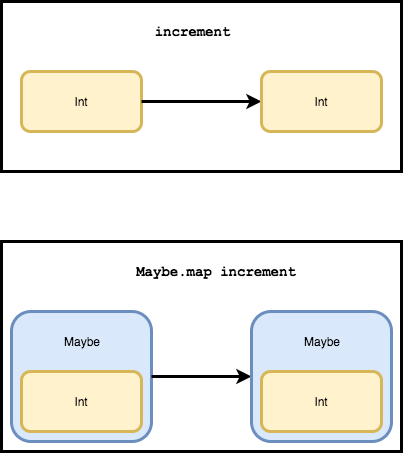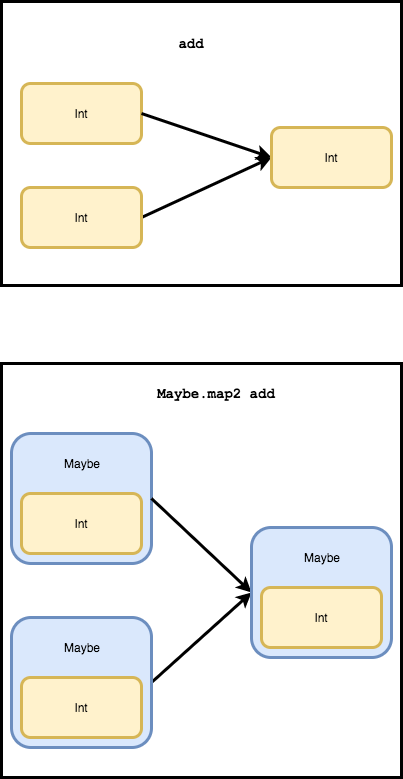# Two ways of looking at map functions

When learning a functional language, you’ll notice that `map` functions are everywhere. Coming from Ruby, I wasn’t familiar with map functions other than `Array#map`.

Now the best way to learn these is to actually use them and get a feel for what they do. After doing that, I noticed that among other things map functions solve two big problems:

1. It’s tedious to wrap/unwrap data structures just to run functions on the values inside them.
2. We need a way to translate “normal” functions we write to work on wrapped values like `Maybe` and `Result`.

If you squint, you may notice that these two problems are actually the same problem viewed from two different angles. So think of this as two different perspectives on mapping functions.

## Problem 1 - Wrapping and unwrapping

You have a wrapper type and want to run a function on the value inside:

``````type Dollar = Dollar Int
``````

Incrementing the dollar value looks like:

``````incrementDollar : Dollar -> Dollar
incrementDollar (Dollar d) =
Dollar (d + 1)
``````

I’m destructuring in the arguments to unwrap the value inside the `Dollar`. That’s a lot of wrapping and unwrapping needed to do something simple.

Trying to double a dollar looks similar:

``````doubleDollar : Dollar -> Dollar
doubleDollar (Dollar d) =
Dollar (d * 2)
``````

in both cases, we’re following the same three steps:

1. Unwrapping the integer
2. Doing something with the integer
3. Re-wrapping the result from step 2

Step 2 is the only part that’s interesting. Steps 1 and 3 are a boilerplate-heavy wrap/unwrap sandwich.

There’s a principle in software development that states:

Separate things that change from things that stay the same.

We can abstract away the wrapping/unwrapping sandwich:

``````mapDollar : (Int -> Int) -> Dollar -> Dollar
mapDollar fn (Dollar d) =
Dollar (fn d)
``````

This looks just like our other two functions but now you can pass it an arbitrary function to use for step 2.

``````incrementDollar : Dollar -> Dollar
incrementDollar dollar =
mapDollar (\d -> d + 1) dollar
``````

### Handling multiple arguments

``````addDollar : Dollar -> Dollar -> Dollar
addDollar (Dollar d1) (Dollar d2) =
Dollar (d1 + d2)
``````

We’re still doing three steps:

1. Unwrap the two integers
2. Calculate something based on the two integers
3. Re-wrap the result of step 2

This is just like our `mapDollar` before but now we work with two arguments.

``````map2Dollar : (Int -> Int -> Int) -> Dollar -> Dollar -> Dollar
map2Dollar fn (Dollar d1) (Dollar d2) =
Dollar (fn d1 d2)
``````

Now we can say:

``````addDollar : Dollar -> Dollar -> Dollar
map2Dollar (+) d1 d2
``````

## Problem 2 - Translating functions

You’ve written some functions for some basic number transformations:

``````increment : Int -> Int
increment n =
n + 1

add : Int -> Int -> Int
n1 + n2
``````

This works fine until you inevitably come across a number wrapped in `Maybe`. Great, now you have to re-implement versions of these functions that work on `Maybe` values. And you’ll probably have to do that again to deal with numbers wrapped in `Result`. If only there was a way to auto-translate your functions.

Well there is!

Notice that the signature of the functions is the same other than possibly being wrapped in `Maybe` or `Result`.

``````increment       :          Int ->          Int -- HAVE
incrementMaybe  : Maybe    Int -> Maybe    Int -- WANT
incrementResult : Result a Int -> Result a Int -- WANT
``````

Enter the `map` function:

``````-- TRANSLATE increment TO WORK ON MAYBE

incrementMaybe : Maybe Int -> Maybe Int
incrementMaybe =
Maybe.map increment
``````
``````-- TRANSLATE increment TO WORK ON RESULT

incrementResult : Result a Int -> Result a Int
incrementResult =
Result.map increment
``````### Multiple arguments

That’s cool but what about our `add` function? It takes two arguments. That’s what `map2` is for (and `map3` for 3-arg functions and so on).

Again, the signatures are similar other than the wrappers:

``````add :                Int ->          Int ->          Int -- HAVE
addMaybe  : Maybe    Int -> Maybe    Int -> Maybe    Int -- WANT
addResult : Result a Int -> Result a Int -> Result a Int -- WANT
``````

Using `map2`, this would look like:

``````-- TRANSLATE add TO WORK ON MAYBE

addMaybe : Maybe Int -> Maybe Int -> Maybe Int
``````
``````-- TRANSLATE add TO WORK ON RESULT

addResult : Result a Int -> Result a Int -> Result a IntEventually some larger patterns emerge. You’ll start seeing how all these perspectives are just that: the same solution viewed from a different angle. With each new perspective on these little functions, you gain a clearer understanding of how where they fit in the world and are better able to see the problems that are best solved with a `map`.